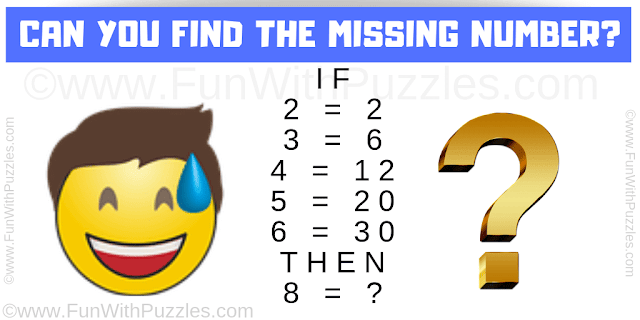This #maths #logic #puzzle is for high school student. This maths logical question will test your logical reasoning and maths skills. In this puzzle, you are provided with some logical number equations. Can you decode the logical reasoning in these given number equation and find the missing number?Can you solve this Maths Logic Puzzle?

Answer of this "Maths Logic Puzzle", can be viewed by clicking on the answer button.